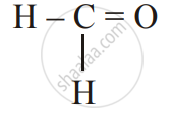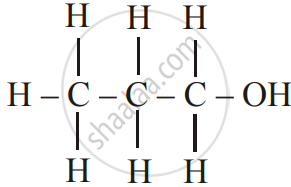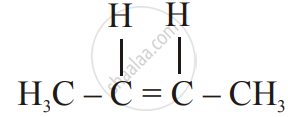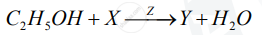# Chemistry Set 1 2017-2018 ICSE Class 10 Question Paper Solution

Chemistry [Set 1]
Date: March 2018

1
1.1 | Choose the correct answer from the options given below :
1.1.1

The salt solution which does not react with ammonium hydroxide is :

Calcium Nitrate

Zinc Nitrate

Copper Nitrate

Concept: Concept of Salts - Types of Salts
Chapter: [0.03] Study of Acids, Bases and Salts
1.1.2

The organic compound which undergoes substitution reaction is :

(A) C_2H_2

(B) C_2H_4

(C) C_10H_18

(D) C_2H_6

Concept: Concept of Organic Compounds
Chapter: [0.09] Organic Chemistry
1.1.3

The electrolysis of acidified water is an example of :

(A) Reduction

(B) Oxidation

(C) Redox reaction

(D) Synthesis

Concept: Electrolysis - Electrolytes and Non-Electrolytes
Chapter: [0.06] Electrolysis
1.1.4

The IUPAC name of dimethyl ether is

(A) Ethoxy methane

(B) Methoxy methane

(C) Methoxy ethane

(D) Ethoxy ethane

Concept: Organic Chemistry - Simple Nomenclature
Chapter: [0.09] Organic Chemistry
1.1.5

The catalyst used in the contact process is

(A) Copper

(B) Iron

(D) Manganese dioxide

Concept: Alcohols - Concept of Ethanol
Chapter: [0.09] Organic Chemistry
1.2 | Give one word or a phase for the following statements :
1.2.1

The energy released when an electron is added to a neutral gaseous isolated atom to form a negatively charged iron.

Concept: Periodic Properties - Electron Affinity
Chapter: [0.01] Periodic Properties and Variations of Properties:- Physical and Chemical
1.2.2

A process of formation of ions from molecules which are not in the ionic state

Concept: Periodic Properties - Ionisation Potential
Chapter: [0.01] Periodic Properties and Variations of Properties:- Physical and Chemical
1.2.3

The tendency of an element to form chains of identical atoms.

Concept: Unique Nature of Carbon Atom
Chapter: [0.09] Organic Chemistry
1.2.4

The property by which certain hydrated salts, when left exposed to the atmosphere, lose their water of crystallization and crumble into powder.

Concept: Concept of Salts
Chapter: [0.03] Study of Acids, Bases and Salts
1.2.5

The process by which sulphide ore is concentrated.

Concept: Extraction of Metals from Ore
Chapter: [0.07] Metallurgy
1.3
1.3.1

Write balanced chemical equations to show : The oxidizing action of conc. Sulphuric acid on carbon

Concept: Sulphuric Acid - Oxidizing Agent When Concentrated
Chapter: [0.084] Sulphuric Acid
1.3.2

Write a balanced chemical equation for the reaction of sodium hydroxide solution with iron (III) chloride solution

Concept: Concept of Acid and Its Properties
Chapter: [0.03] Study of Acids, Bases and Salts
1.3.3

Write a balanced chemical equation for the action of heat on aluminium hydroxide.

Concept: Non Metals and Its Physical Properties
Chapter: [0.07] Metallurgy
1.3.4

Write a balanced chemical equation for the reaction of zinc with potassium hydroxide solution.

Concept: Non Metals and Its Physical Properties
Chapter: [0.07] Metallurgy
1.3.5

Write a balanced chemical equation for Action of dilute hydrochloric acid on magnesium sulphite.

Concept: Concept of Salts
Chapter: [0.03] Study of Acids, Bases and Salts
1.4
1.4.1

Give the IUPAC name for of the following :Concept: Organic Chemistry - Simple Nomenclature
Chapter: [0.09] Organic Chemistry

Give the IUPAC name for of the followingConcept: Organic Chemistry - Simple Nomenclature
Chapter: [0.09] Organic Chemistry

Give the IUPAC name for the followingConcept: Organic Chemistry - Simple Nomenclature
Chapter: [0.09] Organic Chemistry
1.4.2

Write the structural formula of the two isomers of butane

Concept: Organic Chemistry - Simple Nomenclature
Chapter: [0.09] Organic Chemistry
1.5
1.5.1

State one relevant observation for the following.

Lead nitrate solution is treated with sodium hydroxide solution dropwise till it is excess.

Concept: Action of Alkalis (Naoh, Koh) on Certain Metals, Their Oxides and Hydroxides
Chapter: [0.04] Analytical Chemistry:- Use of Ammonium Hydroxide and Sodium Hydroxide
1.5.2

State one relevant observation for the following:

At the anode, when molten lead bromide is electrolyzed using graphite electrodes.

Concept: Electrolysis - Electrolytes and Non-Electrolytes
Chapter: [0.06] Electrolysis
1.5.3

State your observations when Dilute Hydrochloric acid is added to Lead nitrate solution and the mixture is heated.

Concept: Hydrogen Chloride - Acidic Properties of Its Solution
Chapter: [0.083] Hydrogen Chloride
1.5.4

State one relevant observation for the following:

Anhydrous calcium chloride is exposed to air for some time.

Concept: Concept of Salts
Chapter: [0.03] Study of Acids, Bases and Salts
1.5.5

State your observations when Barium chloride solution is mixed with Sodium Sulphate Solution.

Concept: Formation and Colour of Hydroxide Precipitated for Solutions of Salts of Ca, Fe, Cu, Zn and Pb
Chapter: [0.04] Analytical Chemistry:- Use of Ammonium Hydroxide and Sodium Hydroxide
1.6
1.6.1

Why do ionic compounds have high melting points

Concept: Formation and Properties of Ionic Compounds
Chapter: [0.02] Chemical Bonding
1.6.2

Give a reason for Inert gases do not form ions.

Concept: Concept of Coordinate Bond
Chapter: [0.02] Chemical Bonding
1.6.3

Give a reason for Ionisation potential increases across a period, from left to right

Concept: Periodic Properties - Ionisation Potential
Chapter: [0.01] Periodic Properties and Variations of Properties:- Physical and Chemical
1.6.4

Give a reason for Alkali metals are good reducing agents.

Concept: Position of the Metals (Alkali Metals and Alkaline Earth Metals) in the Periodic Table
Chapter: [0.07] Metallurgy
1.6.5

Give a reason for Conductivity of dilute hydrochloric acid is greater than that of acetic acid

Concept: Carboxylic Acids - Concept of Acetic Acid
Chapter: [0.09] Organic Chemistry
1.7
1.7.1

Name the gas that is produced in the given cases :

Sulphur is oxidized by concentrated nitric acid.

Concept: Nitric Acid as an Oxidizing Agent.
Chapter: [0.08199999999999999] Nitric Acid
1.7.2

Name the gas that is produced in the given cases :

An action of dilute hydrochloride acid on sodium sulphide.

Concept: Hydrogen Chloride - Acidic Properties of Its Solution
Chapter: [0.083] Hydrogen Chloride
1.7.3

Name the gas that is produced in the given case:

The action of cold and dilute nitric acid on copper

Concept: Nitric Acid as an Oxidizing Agent.
Chapter: [0.08199999999999999] Nitric Acid
1.7.4

Name the gas that is produced in each of the given case :

At the anode during the electrolysis of acidified water.

Concept: Electrolysis - Electrolytes and Non-Electrolytes
Chapter: [0.06] Electrolysis
1.7.5

Name the gas that is produced in the given case:

The reaction of ethanol and sodium.

Concept: Non Metals and Its Physical Properties
Chapter: [0.07] Metallurgy
1.8 | Fill up the blanks with the correct choice given in brackets.
1.8.1

Ionic or electrovalent compounds do not conduct electricity in their _______ state. (fused/solid)

Concept: Formation and Properties of Ionic Compounds
Chapter: [0.02] Chemical Bonding
1.8.2

Electrolysis of aqueous sodium chloride solution will form ________ at the cathode. (Hydrogen gas / Sodium metal)

Concept: Electrolysis - Electrolytes and Non-Electrolytes
Chapter: [0.06] Electrolysis
1.8.3

Dry hydrogen chloride gas can be collected by ________ displacement of air. (downward / upward)

Concept: Hydrogen Chloride - Acidic Properties of Its Solution
Chapter: [0.083] Hydrogen Chloride
1.8.4

The most common ore of iron is ________. (Calcium / Haematite)

Concept: Metals and Their Alloys - Common Ores of Iron, Aluminium and Zinc
Chapter: [0.07] Metallurgy
1.8.5

The salt prepared by the method of direct combination is _______. (iron (II) chloride / iron (III) chloride)

Concept: Concept of Salts
Chapter: [0.03] Study of Acids, Bases and Salts
2
2.1

What do you understand by a lone pair of electrons?

Concept: Characteristic Properties of Electrovalent Compounds
Chapter: [0.02] Chemical Bonding

Draw the electron dot diagram of Hydionium ion (H = 1; O = 8)

Concept: Characteristic Properties of Electrovalent Compounds
Chapter: [0.02] Chemical Bonding
2.2

In Period 3 of the Periodic Table, element B is placed to the left of element A

On the basis of this information, choose the correct word from the brackets to complete the following statements:

1) The element B would have (lower / higher) metallic character than A

2) The element A would probably have (lesser / higher) electron affinity than B.

3) The element A would have (greater / smaller) atomic size than B.

Concept: Periodic Properties - Metallic Character
Chapter: [0.01] Periodic Properties and Variations of Properties:- Physical and Chemical
2.3

Copy and complete the following table which refers to the conversion of ions to neutral particles.

 Conversion Ionic equation Oxidation / Reduction Chloride ion to chlorine molecule 1)_________ 2)_________ Lead (II) ion to lead 3)_________ 4)_________
Concept: Electrolysis - Elementary Study of the Migration of Ions
Chapter:
3
3.1

Write the balanced chemical equation to prepare ammonia gas in the laboratory by using an alkali.

Concept: Non Metals and Its Physical Properties
Chapter: [0.07] Metallurgy

State why concentrated sulphuric acid is not used for drying ammonia gas

Concept: Non Metals and Its Physical Properties
Chapter: [0.07] Metallurgy

Why is ammonia gas not collected over water?

Concept: Non Metals and Its Physical Properties
Chapter: [0.07] Metallurgy
3.2

Name the acid used for the preparation of hydrogen chloride gas in the laboratory. Why is this particular acid preferred to other acids?

Concept: Hydrogen Chloride - Preparation of Hydrogen Chloride from Sodium Chloride
Chapter: [0.083] Hydrogen Chloride

Write the balanced chemical equation for the laboratory preparation of hydrogen chloride gas.

Concept: Hydrogen Chloride - Preparation of Hydrogen Chloride from Sodium Chloride
Chapter: [0.083] Hydrogen Chloride
3.3

For the preparation of hydrochloric acid in the laboratory:

Why is the direct absorption of hydrogen chloride gas in water not feasible?

Concept: Hydrogen Chloride - Preparation of Hydrogen Chloride from Sodium Chloride
Chapter: [0.083] Hydrogen Chloride

For the preparation of hydrochloric acid in the laboratory:

What arrangement is done to dissolve hydrogen chloride gas in water?

Concept: Hydrogen Chloride - Acidic Properties of Its Solution
Chapter: [0.083] Hydrogen Chloride
3.4

For the electro-refining of copper: What is the cathode made up of?

Concept: Non Metals and Its Physical Properties
Chapter: [0.07] Metallurgy

For the electro-refining of copper: Write the reaction that takes place at the anode

Concept: Non Metals and Its Physical Properties
Chapter: [0.07] Metallurgy
4
4.1

The percentage composition of a gas is Nitrogen 82.35%, Hydrogen 17.64%. Find the empirical formula of the gas.  [N = 14, H = 1]

Concept: Concept of Mole
Chapter: [0.05] Mole Concept and Stoichiometry
4.2

Aluminium carbide reacts with water according to the following equation :

Al_4C_3 + 12H_2O-> 4Al(OH)_3 + 3CH_4

1)What mass of aluminium hydroxide is formed from 12g of aluminium carbide?

2) What volume of methane at s.t.p. is obtained from 12g of aluminium carbide?

[Relatively molecular weight of Al_4Cl_3 = 144; Al(OH)_3 = 78]

Concept: Vapour Density and Its Relation to Relative Molecular Mass:
Chapter: [0.05] Mole Concept and Stoichiometry
4.3

If 150 cc of gas A contains X molecules, how many molecules of gas B will be present in 75 cc of B?. The gases A and B are under the same conditions of temperature and pressure.

Chapter: [0.05] Mole Concept and Stoichiometry

Name the law om which same conditions of temperature and pressure

Chapter: [0.05] Mole Concept and Stoichiometry
4.4

State the main components of the following alloys:

Brass

Concept: Metals and Their Alloys - Common Ores of Iron, Aluminium and Zinc
Chapter: [0.07] Metallurgy

State the main components of the following alloys:

Duralumin

Concept: Metals and Their Alloys - Common Ores of Iron, Aluminium and Zinc
Chapter: [0.07] Metallurgy
5
5.1

Complete the following table which relates to the homologous series of hydrocarbons.

 General Formula IUPAC name of the homologous series Characteristic bond type IUPAC name of the first member of the series C_nH_(2n-2) (A)________ (B)______ (C)______ C_nH_(2n+1) (B)________ (E)______ (F)______
Concept: Versatile Nature of Carbon - Homologous Series of Carbon Compound
Chapter: [0.09] Organic Chemistry
5.2

Name the most common ore of the metal aluminium from which the metal is extracted. Write the chemical formula of the ore.

Concept: Metals and Their Alloys - Common Ores of Iron, Aluminium and Zinc
Chapter: [0.07] Metallurgy

Name the process by which impure ore of aluminium gets purified by using the concentrated solution of an alkali.

Concept: Metals and Their Alloys - Common Ores of Iron, Aluminium and Zinc
Chapter: [0.07] Metallurgy

Write the equation for the formation of aluminium at the cathode during the electrolysis of alumina.

Concept: Metals and Their Alloys - Common Ores of Iron, Aluminium and Zinc
Chapter: [0.07] Metallurgy
6
6.1

A compound X (having the vinegar-like smell) when treated with ethanol in the presence of the acid Z, gives a compound Y which has a fruity smell.

The reaction is:1) Identify Y and Z.

2) Write the structural formula of X.

3) Name the above reaction.

Concept: Action of Alkalis (Naoh, Koh) on Certain Metals, Their Oxides and Hydroxides
Chapter: [0.04] Analytical Chemistry:- Use of Ammonium Hydroxide and Sodium Hydroxide
6.2

Ethane burns in oxygen to form CO2 and H2O according to the equation:

2C_2H_6+7O_2 -> 4CO_2 + 6H_2O

If 1250 cc of oxygen is burnt with 300 cc of ethane.

Calculate:

1) the volume of CO_2 formed

2) the volume of unused O_2

Concept: Deduction of Simple (Empirical) and Molecular Formula from the Percentage Composition of a Compound
Chapter: [0.05] Mole Concept and Stoichiometry
6.3

Three solutions P, Q and R have pH value of 3.5, 5.2 and 12.2 respectively. Which one of these is a:

1) Weak acid?

2) Strong alkali?

Concept: Concept of Acid and Its Properties
Chapter: [0.03] Study of Acids, Bases and Salts
7
7.1

Give a chemical test to distinguish between the given pairs of chemicals:

Lead nitrate solution and Zinc nitrate solution

Concept: Action of Alkalis (Naoh, Koh) on Certain Metals, Their Oxides and Hydroxides
Chapter: [0.04] Analytical Chemistry:- Use of Ammonium Hydroxide and Sodium Hydroxide

Give a chemical test to distinguish between the given pairs of chemicals:

Sodium chloride solution and Sodium nitrate solution

Concept: Hydrogen Chloride - Acidic Properties of Its Solution
Chapter: [0.083] Hydrogen Chloride
7.2

Write a balanced equation for the preparation of the following salts:

Copper sulphate from Copper Carbonate.

Concept: Concept of Salts
Chapter: [0.03] Study of Acids, Bases and Salts

Write a balanced equation for the preparation of the following salts:

Zinc carbonate from Zinc sulphate

Concept: Concept of Salts
Chapter: [0.03] Study of Acids, Bases and Salts
7.3

What is the type of salt formed when the reactants are heated at a suitable temperature for the preparation of Nitric acid?

Concept: Nitric Acid - Laboratory Method of Preparation of Nitric Acid from Potassium Nitrate Or Sodium Nitrate
Chapter: [0.08199999999999999] Nitric Acid
7.4

Which property of sulphuric acid is shown by the reaction of the concentrated sulphuric acid with Ethanol?

Concept: Sulphuric Acid - Oxidizing Agent When Concentrated
Chapter: [0.084] Sulphuric Acid

Which property of sulphuric acid is shown by the reaction of the concentrated sulphuric acid with Carbon?

Concept: Sulphuric Acid - Oxidizing Agent When Concentrated
Chapter: [0.084] Sulphuric Acid

#### Request Question Paper

If you dont find a question paper, kindly write to us

View All Requests

#### Submit Question Paper

Help us maintain new question papers on Shaalaa.com, so we can continue to help students

only jpg, png and pdf files

## CISCE previous year question papers Class 10 Chemistry with solutions 2017 - 2018

CISCE Class 10 Chemistry question paper solution is key to score more marks in final exams. Students who have used our past year paper solution have significantly improved in speed and boosted their confidence to solve any question in the examination. Our CISCE Class 10 Chemistry question paper 2018 serve as a catalyst to prepare for your Chemistry board examination.
Previous year Question paper for CISCE Class 10 Chemistry-2018 is solved by experts. Solved question papers gives you the chance to check yourself after your mock test.
By referring the question paper Solutions for Chemistry, you can scale your preparation level and work on your weak areas. It will also help the candidates in developing the time-management skills. Practice makes perfect, and there is no better way to practice than to attempt previous year question paper solutions of CISCE Class 10.

How CISCE Class 10 Question Paper solutions Help Students ?
• Question paper solutions for Chemistry will helps students to prepare for exam.
• Question paper with answer will boost students confidence in exam time and also give you an idea About the important questions and topics to be prepared for the board exam.
• For finding solution of question papers no need to refer so multiple sources like textbook or guides.